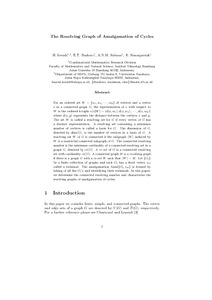# The Resolving Graph of Amalgamation of Cycles

Iswadi, Hazrul and Baskoro, Edy Tri and Salman, A.N.M and Simanjuntak, Rinovia (2010) The Resolving Graph of Amalgamation of Cycles. Utilitas Mathematica, 83. pp. 121-132. ISSN 0315-3681Preview PDF hazrul_The Resolving Graph of Amalgamation of Cycles_2010.pdf Download (173kB) | Preview

## Abstract

For an ordered set W = {w_1,w_2,...,w_k} of vertices and a vertex v in a connected graph G, the representation of v with respect to W is the ordered k-tuple r(v|W) = (d(v,w_1),d(v,w_2),...,d(v,w_k)) where d(x,y) represents the distance between the vertices x and y. The set W is called a resolving set for G if every vertex of G has a distinct representation. A resolving set containing a minimum number of vertices is called a basis for G. The dimension of G, denoted by dim(G), is the number of vertices in a basis of G. A resolving set W of G is connected if the subgraph <W> induced by W is a nontrivial connected subgraph of G. The connected resolving number is the minimum cardinality of a connected resolving set in a graph G, denoted by cr(G). A cr-set of G is a connected resolving set with cardinality cr(G). A connected graph H is a resolving graph if there is a graph G with a cr-set W such that <W> = H. Let {G_i} be a finite collection of graphs and each G_i has a fixed vertex v_{oi} called a terminal. The amalgamation Amal{Gi,v_{oi}} is formed by taking of all the G_i's and identifying their terminals. In this paper, we determine the connected resolving number and characterize the resolving graphs of amalgamation of cycles.

Item Type: Article Resolving Graph, Resolving Set, Amalgamation, Cyacles Q Science > QA Mathematics Academic Department > Department of Mathematics and Natural Science Hazrul Iswadi 6179 08 Mar 2012 07:04 20 Mar 2012 01:41 http://repository.ubaya.ac.id/id/eprint/173View Item